Pip Counting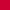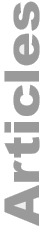The Ash Dalvi 13 Count, Part 1 Ashutosh DalviMarch 2011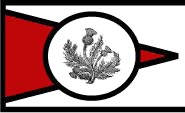Introduction

I am not good at mental arithmetic. So, I have tried to devise something easier.

I noticed that in almost every pip count, I would be multiplying by 13 at some stage due to checkers on the mid point. This gave me an idea. I'm calling it The Ash Dalvi 13 Count. The idea is that it is easier to do one multiplication by 13 rather than adding a bunch of big numbers together.

What you need

You need to learn your 13 times table up to at least 13 × 10. If you need a higher product such as 13 × 16, you can just do 13 × 6 = 78 and add this to 130 (13 × 10). However, it's better to just learn the 13 times table up to about 20. The less steps involved in counting, the less chance of making a mistake and faster you will count.

 13 × 1 = 13 13 × 2 = 26 13 × 3 = 39 13 × 4 = 52 13 × 5 = 65 13 × 6 = 78 13 × 7 = 91 13 × 8 = 104 13 × 9 = 117 13 × 10 = 130 13 × 11 = 143 13 × 12 = 156 13 × 13 = 169 13 × 14 = 182 13 × 15 = 195 13 × 16 = 208 13 × 17 = 221 13 × 18 = 234 13 × 19 = 247 13 × 20 = 260

You need to familiarise yourself with the following 3 positions. The idea is that checkers close to these positions will be counted as actually being in these positions and at the end, you do what is called a mental shift (i.e., you correct for the difference compared to these positions).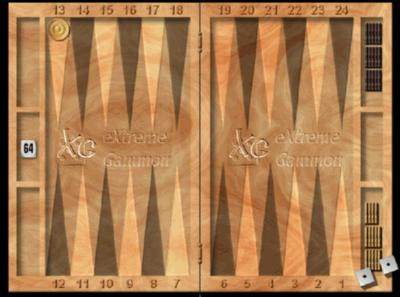Position A.A checker on the 13 pt counts as one 13.

Simplicity is the key and things don't get much simpler than being told a checker on the 13 pt counts as one 13.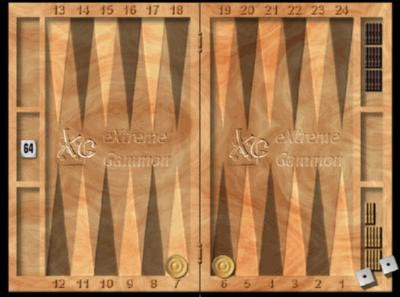Position B.Two checkers equidistant from your bar counts as one 13.

Two checkers equidistant from your bar count as one 13. Therefore, if you have an even number of checkers around the bar, divide the number of checkers by 2 to get your 13 count.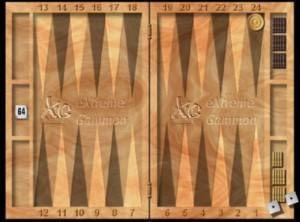Position C.A checker in your opponent's home board or on the bar is equivalent to two 13s.

No, I haven't made a mistake in adding. You can do a simple correction at the end.

The simple method to correct for counting the checkers in your opponent's home board as two 13s is as follows.

1. From the 13 count subtract the number of checkers in your opponent's home board.

2. Then subtract the pipcount of the checkers counting the pipcount in terms of your opponent's board points. (I.e., your 24 pt is your opponent's 1 point and will count as 1 pip; your 23 point will count as 2 pips; your 22 pt will count as 3 pips; the bar counts as 0 etc.)

So, in the above case you would have two 13s (26), minus number of checkers (1), minus pipcount from the perspective of your opponent's home board board points (1), equals 24.

The Method

The method involves counting the 13s and then doing mental shifts. The checkers do not have to be exactly in these positions—they just have to be close.

Example 1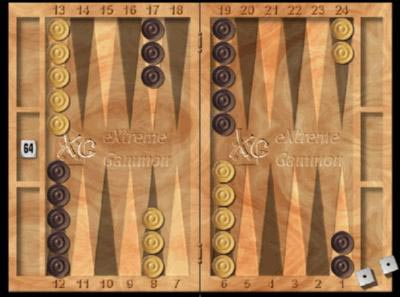Step 1. Figure out your strategy as to how you will count the 13s.

• The 5 checkers on the 13 pt can be counted as normal 13s as in Position A.
5 on the 13 pt (Position A) = 5 sets of 13.

• The 8 around the bar can be counted checkers equidistant from the bar as in Position B.
8 divide 2 = 4 sets of 13 (Position B).

• The 2 checkers on the 24 pt can be counted as checkers in your opponent's home board as in Position C.
2 checkers on the 24 pt (Position C) = 4 sets of 13.

• That adds up to a 13 count of 13 − 13 × 13 = 169 pips.

Step 2. Mental shifts.

• 13 pt (Position A): No mental shifts required as all checkers are on the 13 pt.

• Equidistant from your bar (Position B): To get 4 at an equal distance either side of the bar, you need to move the 3 on the 8 pt to the 7 pt (+3) and 1 from the 6 pt to the 7 pt (-1). This comes to 2 pips to add onto your total. 169 + 2 = 171.

• Checkers in your opponent's home board (Position C): The number of checkers in your opponent's home board is 2. The pipcount (in terms of your opponent's home board points) of the checkers in your opponent's home board is 2. Subtract these from the running total: 171 &minus 2 &minus 2 = 167.

Example 2

Let's try a more complicated example.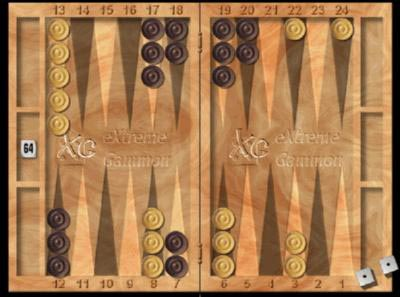White's 13 Count:

Step 1. Figure out your strategy as to how you will count the 13s.

• I will ignore the checkers on the 3 pt and just add them on at the end.

• The 5 checkers on the 13 pt can be counted as normal 13s as in Position A. Five 13s.

• The 6 around the bar can be counted as equidistant from the bar as in Position B. Six checkers on the 8 and 6 pts = three 13s.

• The checkers on the 22 and 24 pts count as checkers in your opponent's home board (Position C). Two checkers in your opponent's home board count as four 13s.

• The 13 count is 12. 12 × 13 = 156.

Step 2. Mental shifts.

• 13 pt (Position A): No shift needed.

• Checkers equidistant from the bar (Position B): You need to shift 3 from the 8 pt to the 7 pt (+3). So the total is now 159.

• Checkers in your opponent's home board (Position C): Running total (159), minus number of checkers (2), minus pips in terms of your opponent's home board points (4), equals 153.

• Now, add the 6 pips for the checkers on the 3 point: 153 + 6 = 159.

Black's 13 count:

Step 1. Figure out your strategy as to how you will count the 13s.

• 13 pt (Position A): I will count the 18 pt checkers as 13 pt checkers. So, five 13s.

• The 10 around the bar can be counted as in Position B: 10 checkers is five 13s. So we have a 13 count of 10. 13 × 10 = 130.

Step 2. Mental shifts.

• 13 pt (Position A): The 2 on the 18 pt should be on the 13 pt (+10) Total is 130 + 10 = 140.

• Checkers around the bar (Position B): You only have to make 1 shift to make all checkers equidistant from the bar. Move one checker from the 8 to the 7 pt (+1). Grand total is 140 + 1 = 141.

Another PositionPosition D.Two opposite checkers + 1 pip equals 2 sets of 13.

This gives you another way of counting a checker in your opponent's home board if you can pair it with a checker in your home board.

If you look at Example 2 above, the 2 checkers on white's 3 point can be paired with the 2 checkers in his opponent's home board. If you were to just count these 4 checkers, your 13 count would be 2 for each opposite pair which would add up to 4. In order to correct for the checkers in white's opponent's home board not being on his 2 point, you would have to move 1 checker back from the 22 point to the 23 point (−1) and 1 checker forward from the 24 point to the 23 point (+1). This adds up to zero and so there would be no correction. The 4 checkers add up to (13 × 4) 52 pips.

Other frequent uses for Position D include:

• Pairing a 20 point anchor with an equal number of checkers on your 6 point.
• Pairing an 18 point anchor with an equal number of checkers on your 8 point.

There are only 4 key positions to memorise.

The 15 checkers on the board can often be treated as a giant cluster. Sometimes you may not get all the checkers into the 13 count. However, it is usually one or two checkers that are on low points that are easy to add on at the end.

The method has advantages over standard cluster counting (although I do think cluster counting has its usefulness in certain situations). With cluster counting, you add the big numbers together and you still make mental shifts if the checkers are not precisely in the right place. The Ash Dalvi method actually includes the step of multiplying by 13 in exchange for adding big numbers together. If you learn your 13 times table by heart, you are less likely to make a mistake compared to adding big numbers together. It will also take less time.

If all you need to know is who is ahead in the race, you can count the 13s in seconds. If you are two 13s ahead, you are almost sure to be ahead after the mental shifts. If you are one 13 ahead, you are still likely to be ahead but you might have to do the mental shifts.

Generally, it seems like a natural method. You start with a bunch of checkers on the 13 pt and a cluster around the bar. Furthermore, general backgammon strategy involves keeping the 13 pt and trying to build points around your bar. So, you have to multiply by 13 in most positions anyway.

Other Tips

You can count an 18 point checker as a 13; it has a correction of +5.

You can count an 8 point checker as a 13; it has a correction of −5.

Example 3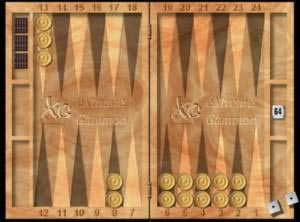Once most of the checkers are in the home board, I often combine my method with cluster counting. I can see the 5 prime is 40 pips. So, I will count all 5 checkers outside my home board as 13s and subtract 10 to correct for the 2 checkers on the 8 pt.

13 × 5 = 65 − 10 = 55 + 40 (for the 5 prime with a middle point of 4) = 95.

Easy peasy!

Don't always break your 10s into 13s. If I have a 5 prime, I know the middle point multiplied by 10 is the total pip count in the prime. I will add this on to the 13 total.

In the next article, I will discuss how you might incorporate checkers in your home board into the 13 count.

With a little practice, you can count some seemingly complicated positions relatively effortlessly in just a few seconds. You might even find that pip-counting is almost fun.